Students can Download Tamil Nadu 12th Chemistry Model Question Paper 2 English Medium Pdf, Tamil Nadu 12th Chemistry Model Question Papers helps you to revise the complete Tamilnadu State Board New Syllabus, helps students complete homework assignments and to score high marks in board exams.

## TN State Board 12th Chemistry Model Question Paper 2 English Medium

Time: 3 Hours
Maximum Marks: 70

Instructions:

1. The question paper comprises of four parts
2. You are to attempt all the parts An internal choice of questions is provided wherever applicable
3. All questions of Part I, II, III, and IV are to be attempted separately
4. Question numbers 1 to 15 in Part I are Multiple Choice Questions of one mark each. These are to be answered by choosing the most suitable answer from the given four alternatives and writing the option code and the corresponding answer
5. Question numbers 16 to 24 in Part II are two-mark questions. These are lo be answered in about one or two sentences
6. Question numbers 25 to 33 in Part III are three-marks questions. These are to be answered in about three to five short sentences
7. Question numbers 34 to 38 in Part IV are five-mark Questions These are to answered in detail. Draw diagrams wherever necessary

Part – 1

Answer all the questions. Choose the correct answer. [15 × 1 = 15]

Question 1.
Blistered copper is .
(a) 98% pure copper
(b) 96% pure copper
(c) 97% pure copper
(d) 88% pure copper
(a) 98% pure copper

Question 2.
Which of the following statements is not correct?
(a) Beryl is a cyclic silicate
(b) Mg2SiO4 is an orthosilicate
(c) SiO4 is the basic structural unit of silicates
(d) Feldspar is not aluminosilicate
(d) Feldspar is not aluminosilicate

Question 3.
Consider the following statements.
(i) phosphine is the most important hydride of phosphorous
(ii) phosphine is a poisonous gas with rotten egg smell.
(iii) phosphine is a powerful reducing agent
Which of the above statement(s) is / are correct?
(a) (i) and (ii)
(b) (ii) and (iii)
(c) (i) and (iii)
(d) (ii) only
(c) (i) and (iii)Question 4.
The correct order of increasing oxidizing power in the series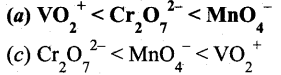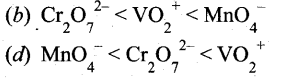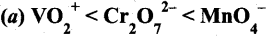Hint: VO2+ < Cr2O72- < MnO4 greater the oxidation state, higher is the oxidising power.

Question 5.
The geometry possible in [Fe F6]4- and [COF+6]4- is ……………
(a) Trigonal bipyramidal
(b) Square planar
(c) Octahedral
(d) Tetrahedral
(c) Octahedral

Question 6.
The number of carbon atoms per unit cell of diamond is
(a) 8
(b) 6
(c) 1
(d) 4
(a) 8

Hint: In diamond carbon forming fee. Carbon occupies comers and face centres and also occupying half of the tetrahedral voids.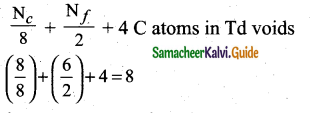Question 7.
In a homogeneous reaction
A → B + C + D, the initial pressure was P0 and after time t it was P. Expression for rate constant in terms of P0 , P and t will be ……..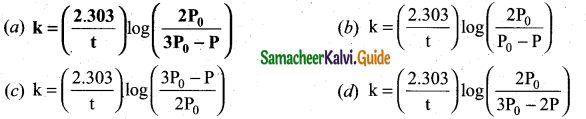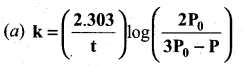Solution: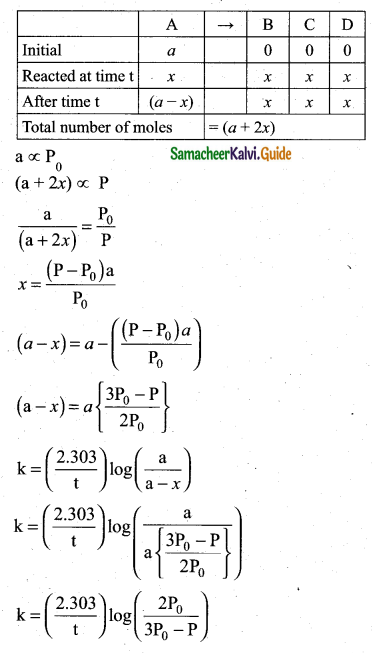Question 8.
The solubility of an aqueous solution of Mg(OH)2 be x then its Ksp is
(a) 4x3
(b) 108x5
(c) 27x4
(d) 9x
(a) 4x3
Solution:
Mg(OH)2 Mg2+(x) + 2OH ((2x)2)
Ksp = 4x3Question 9.
During electrolysis of molten sodium chloride, the time required to produce 0. lmol of chlorine gas using a current of 3 A is ……………..
(a) 55 minutes
(b) 107.2 minutes
(c) 220 minutes
(d) 330 minutes
Hint: m = ZIt (mass of 1 mole of Cl2 gas = 71)
(b) 107.2 minutes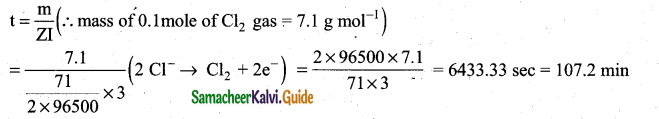Question 10:
The coagulation values in millimoles per litre of the electrolytes used for the coagulation of AS2S3 are given below
(I) (NaCl) = 52
(II) (BaCl) = 0.69
(III) (MgSO4) = 0.22
The correct order of their coagulating power is
(a) III > II > I
(b) I > II > III
(c) I > III > II
(d) II > III> I
coagulating power ± $$\frac{1}{\text { coagulation value }}$$
(a) III > II > IQuestion 10.
Assertion : Coagulation power of Al3+ is more than Na+ .
Reason : greater the valency of the flocculating ion added, greater is its power to cause precipitation
(a) if both assertion and reason are true and reason is the correct explanation of assertion. (Hardy-Sechulze rule)
(b) if both assertion and reason are true but reason is not the correct explanation of assertion.
(c) assertion is true but reason is false
(d) both assertion and reason are false.
(a) if both assertion and reason are true and reason is the correct explanation of assertion. (Hardy-Sechulze rule)

Question 11.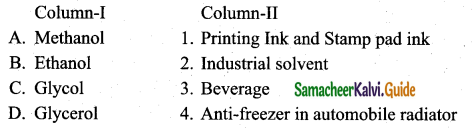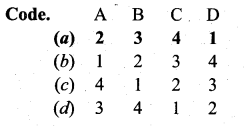(a) A- 2, B – 3, C – 4, D – 1

Question 12.
Which of the following represents the correct order of acidity in the given compounds (
(a) FCH2COOH > CH3COOH > BrCH2COOH > ClCH3COOH
(b) FCH2COOH > ClCH2COOH > BrCH COOH > CH COOH
(c) CH3COOH > ClCH2COOH > FCH2COOH > Br – CH2COOH
(d) Cl CH2COOH > CH3COOH > BrCH2COOH > ICH2COOH
Hint. -I effect increases the acidity. If electronegativity is high, -I effect is also high.
(b) FCH2COOH > ClCH2COOH > BrCH COOH > CH COOH

Question 13.
1 -nitrobutane and 2-methyl-1 -nitropropane are belong to
(a) position isomerism
(b) functional isomerism
(c) Tautomerism
(d) chain isomerism
(d) chain isomerism

Question 14.
Haemoglobin is
(a) an enzyme
(b) a globular protein
(c) a vitamin
(d) carbohydrate
(b) a globular protein

Question 15.
An example of antifertility drug is
(a) novestrol
(b) seldane
(c) salvarsan
(d) Chloramphenicol
(a) novestrol

Part – II

Answer any six questions. Question No. 20 is compulsory. [6 x 2 = 12]

Question 16.
Name the method used for the refining of (i) Nickel (ii) Zirconium
(i) Mond’s process
(ii) Van Arkel’s method

Question 17.
Complete the following reactions:
(a) B(OH)3 + NH3
(b) Na2B4O7 + H2SO4 + H2O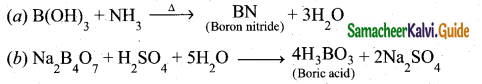Question 18.
KMnO4 does not act as oxidising agent in the presence of HC1. Why?
HCl cannot be used for making acidified KMnO4 as oxidising agent, since it reacts with KMnO4 as follows.
2MnO4 + 10 Cl + 16H+ → 2Mn2+ + 5Cl2 + 8H2O

Question 19.
A reaction is first order in A and second order in B.
(i) Write the differential rate equation.
(ii) How is the rate affected on increasing the concentration of B three times?
(iii) How is the rate affected when the concentrations of both A and B are doubled? ,
(i) $$\frac{d x}{d t}=k[\mathrm{A}]^{1}[\mathrm{B}]^{2}$$
(ii) If concentration of ‘B ’ is tripled, then the rate will become 9 times.
(iii) When concentration of both A and B are doubled, then the rate will become 8 times.

Question 20.
Calculate the pH of 0.04 M HNO3 Solution.
Concentration of HNO3 = 0.04M
[H3O+] = 0.04 mol dm-3
pH = -log[H3O+]
= – log(0.04)
= – log(4 x 10-2)
= 2 -log 4 = 2-0.6021
= 1.3979= 1.40

Question 21.
Write a note about Helmoholtz double layer.
The surface of a colloidal particle adsorbs one type of ion due to preferential adsorption. This layer attracts the oppositely charged ions in the medium and hence the boundary separating the two electrical double layers are set up. This is called Helmholtz electrical double layer. As the particles nearby are having similar charges, they cannot come close and condense. Hence this helps to explain the stability of the colloid.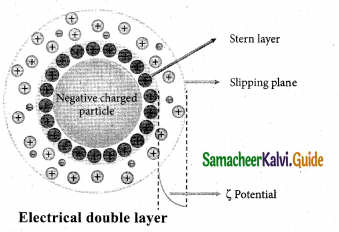Question 22.
Why HCOOH does not give Hell-Volhard Zelinsky reaction but CH33COOH does?
CH3COOH contains α – hydrogen atom and hence gives HVZ reaction but HCOOH does not contain an α – hydrogen atom and hence does not give HVZ reaction.

Question 23.
What happens when
Oxidation of acetoneoxime with trifluoroperoxy acetic acid.
Oxidation of acetoneoxime with trifluoroperoxy acetic acid gives 2-nitropropane.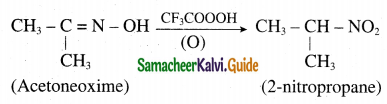Question 24.
What are food preservatives?
Food preservatives are chemical substances are capable of inhibiting, retarding or arresting the process of fermentation, acidification or other decomposition of food by growth of micro organisms.
Examples:

• Acetic acid is used mainly as a preservative for preparation of pickles.
• Sodium meta sulphite is used as preservative for fresh vegetables and fruits.
• Sodium benzoate is used as preservative for juices.

Part – III

Answer any six questions. Question No. 31 is compulsory. [6 x 3 = 18]

Question 25.
Explain the principle of electrolytic refining with an example.
The crude metal is refined by electrolysis. It is carried out in an electrolytic cell containing aqueous solution of the salts of the metal of interest. The rods of impure metal are used as anode and thin strips of pure metal are used as cathode. The metal of interest dissolves from the anode, pass into the solution while the same amount of metal ions from the solution will be deposited at the cathode. During electrolysis, the less electropositive impurities in the anode, settle down at the bottom and are removed as anode mud.
Let us understand this process by considering electrolytic refining of silver as an example.
Cathode: Pure silver
Anode: Impure silver rods

Electrolyte: Acidified aqueous solution of silver nitrate.
When a current is passed through the electrodes the following reactions will take place
Reaction at anode: 2Ag(s) → Ag+ (aq) + le
Reaction at cathode: Ag+(aq)+ le → Ag (s)

During electrolysis, at the anode the silver atoms lose electrons and enter the solution. The positively charged silver cations migrate towards the cathode and get discharged by gaining electrons and deposited on the cathode. Other metals such as copper, zinc etc.,can also be refined by this process in a similar manner.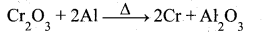Question 26.
How will you prepare phosphine and explain the purification of phosphine?
Phosphine’is prepared by action of sodium hydroxide with white phosphorous in an inert atmosphere of carbon dioxide or hydrogen.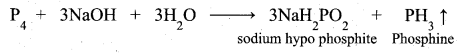Phosphine is freed from phosphine dihydride (P2H4) by passing through a freezing mixture. The dihydride condenses while phosphine does not.
Phosphine can also prepared by the hydrolysis of metallic phosphides with water or dilute mineral acids.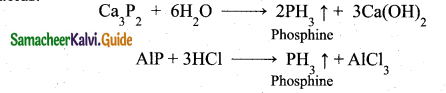Phosphine is prepared in pure form by heating phosphorous acid.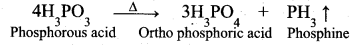A pure sample of phosphine is prepared by heating phosphonium iodide with caustic soda S0lution.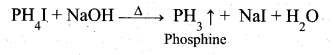Question 27.
Justify the position of lanthanides and actinides in the periodic table.
(i) In sixth period after lanthanum, the electrons are preferentially filled in inner 4f sub shell and these 14 elements following lanthanum show similar chemical properties. Therefore these elements are grouped together and placed at the bottom of the periodic table. This position can be justified as follows.
(a) Lanthanoids have general electronic configuration [Xe] 4f2 14 5d° 1 6s2
(b) The common oxidation state of lanthanoids is +3
(c) All these elements have similar physical and chemical properties.

(ii) Similarly the fourteen elements following actinium resemble in their physical and chemical properties.

(iii) If we place these elements after Lanthanum in the periodic table below 4d series and actinides below 5d series, the properties of the elements belongs to a group would be different and it would affect the proper structure of the periodic table.

(iv) Hence a separate position is provided to the inner transition elements at the bottom of the periodic table.Question 28.
Distinguish between isotropy and anisotropy?
Isotropy:

1. Isotropy means uniformity in all directions.
2. Isotropy means having identical values of physical properties such as refractive index, electrical conductance in all directions.
3. Isotropy is the property of amorphous solids.

Anisotropy :

1. Anisotropy means non-uniformity in all directions.
2. Anisotropy is the property which depends on the direction of measurement. They show different values of physical properties when measured along different directions.
3. Anisotropy is the properly of crystalline solids.

Question 29.
What are the merits and limitations of the intermediate compound theory?
Merits:
(i) The specificity of a catalyst
(ii) The increase in the rate of the reaction with increase in the concentration of catalyst.

Limitations:
(i) This theory fails to explain the action of catalytic poison and promoters. .
(ii) This theory is unable to explain the mechanism of heterogeneous catalysed reactions.

Question 30.
Write a note on sacrificial protection.
In this method, the metallic structure to be protected is made cathode by connecting it with more active metal (anodic metal). So that all the corrosion will concentrate only on the active metal. The artificially made anode thus gradually gets corroded protecting the original metallic structure. Hence this process is otherwise known as sacrificial anodic protection.
Al, Zn and Mg are used as sacrificial anodes.

Question 31.
1mole of Hlis allowed to react with t-butyl methylether. Identify the product and write down the mechanism of the reaction.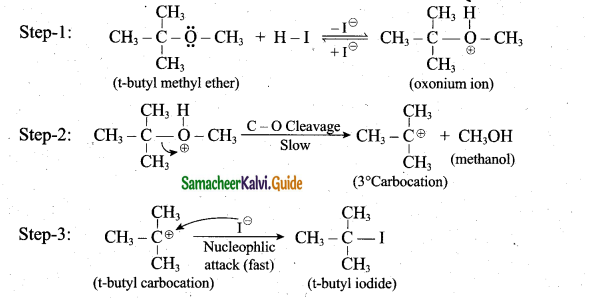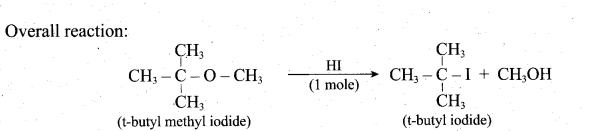Question 32.
How will you prove the presence of aldehyde group in glucose?
(i) Glucose is oxidised to gluconic acid with ammoniacal silver nitrate (Tollen’s reagent) and alkaline copper sulphate (Fehling’s solution). Tollen’s reagent is reduced to metallic silver and Fehling’s solution to cuprous oxide (red precipitate). These reactions confirm the presence of an aldehye group in glucose.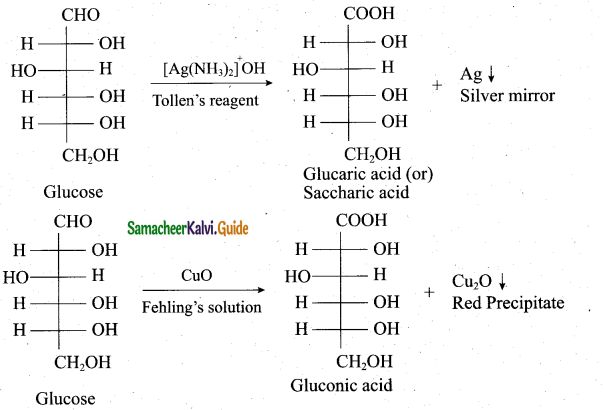Question 33.
Explain about anaesthetics with their types.
(i) Local anaesthetics: It causes loss of sensation in the area in which it is applied without losing consciousness. They block pain perception that is transmitted via peripheral nerve fibres to the brain.
Example: Procaine, Li do caine.
They are often used during minor surgical procedures.

(ii) General anaesthetics: They cause a controlled and reversible loss of consciousness by affecting central nervous system.
Example: Propofol, Isoflurane.
They are often used for major surgical procedures.

Part – IV

Answer all the questions. [5 x 5 = 25]

Question 34.
(a) (i) CO is more stable at higher temperature. Why? (2)
(ii) How will you prepare potash alum? (3)
[OR]
(b) (i) Give the balanced equation for the reaction between chlorine with cold NaOH. (3)
(ii) Nitrogen exists as diatomic molecule and Phosphrus as P4 Why? (2)
(a) (i) In the Ellingham diagram, the formation of carbon monoxide is a straight line with negative slope. In this case AS is positive as 2 moles of CO gas is formed by the consumption of one mole of oxygen. Hence, CO is more stable at higher temperature.

(ii) The alunite the alum stone is the naturally occurring form and it is K2SO4 . Al2(S04)3.4Al(0H)3. When alum stone is treated with excess of sulphuric acid, the aluminium hydroxide is converted to aluminium sulphate. A calculated quality of – potassium sulphate is added and the solution is crystallised to generate potash alum. It is purified by recrystallisation.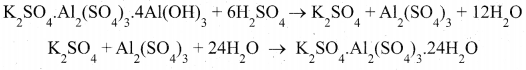(2)

(b)(i) Reaction between chlorine with cold NaoH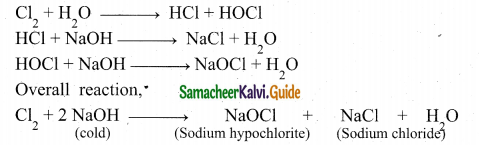Chlorine reacts with cold NaOH to give sodium chloride and sodium hypochlorite.

(ii) Nitrogen has a triple bond between its two atoms because of its small size and high electronegativity. Phosphorus P4 has single bond, that is why it is tetra-atomic.

Question 35.
(a) (i) Discuss the ortho and pyro silicates. (2)
(ii) Compare lanthanides and actinides. (3)
[OR]
(b) (i) Ni2+ is identified using alcoholic solution of dimethyl glyoxime. Write the structural formula for the rosy red precipitate of a complex formed in the reaction. (3)
Cu+, Zn2+, Sc3+, Ti4+ are colourless. Prove this statement. (2)
Ortho silicates: The simplest silicates which contain discrete [SiO4]4- tetrahedral units are called ortho silicates or neso silicates.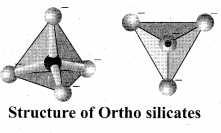Examples: Phenacite – Be2SiO4(Be2+ ions are tetrahedrally surrounded by O2- ions), Olivine – (Fe/Mg)2 SiO4 (‘Fe2+ and Mg2+ cations are octahedrally surrounded by O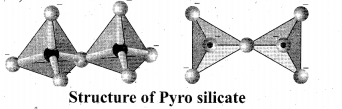Pyro silicates: Silicates which contain [Si2O7]6- ions are called pyro silicates (or) Soro silicates. They are formed by joining two [SiO4]4-

tetrahedral units by sharing one oxygen atom at one comer, (one oxygen is removed while joining). Example: Thortveitite – Sc2Si2O7

(ii)
Lanthanoids

1. Differentiating electron enters in 4f orbital
2. Binding energy of 4f orbitals are higher
3. They show less tendency to form complexes
4. Most of the lanthanoids are colourless
5. They do not form oxo cations
6. Besides +3 oxidation states lanthanoids show +2 and +4 oxidation states in few cases.

Actinoids

1. Differentiating electron enters in 5f orbital
2. Binding energy of 5f orbitals are lower
3. They show greater tendency to form complexes
4. Most of the actinoids are coloured.
5. E.g : U3+ (red), U4+ (green), UO22+ (yellow)
6. They do form oxo cations such as UO22+ NpO22+ etc.
7. Besides +3 oxidation states actinoids show higher oxidation states such as +4, +5, +6 and +7.

[OR]

(b) (i) Ni2+ ions present in Nickel chloride solution is estimated accurately for forming an insoluble complex called [Ni(DMG)2]
Nickel ion reacts with alcoholic solution of DMG in the presence of ammonical medium, to give rosy red precipitate of [Ni(DMG)2] complex.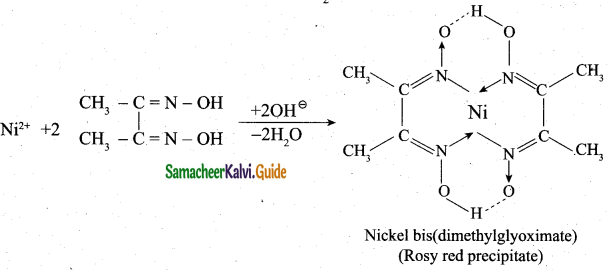(ii) 1. Cu+, Zn2+ have d10 configuration and Sc3+, Ti4+have d1 configuration.
2. d-d transition is not possible in the above complexes. So they are colourless.Question 36.
(a) i)0 What is meant by the term “coordination number”? What is the coordination number of atoms in a bcc structure? (2)
(ii) Rate constant k of a reaction varies with temperature T according to the following Arrhenius equation
$$\log \mathbf{k}=\log \mathbf{A} \frac{\mathbf{E}_{\mathbf{a}}}{2.303 \mathbf{R}}\left(\frac{1}{\mathbf{T}}\right)$$

Where Ea is the activation energy. When a graph is plotted for logk Vs $$\frac{1}{T}$$ a straight line
with a slope of- 4000K Is obtained. Calculate the activation energy. (3)

(OR)

(b) (i) Explain about the hydrolysis of salt of strong base and weak acid. Derive the value of Kh for that reaction. (3)
(ii) Identify the Lewis acid and the Lewis base in the following reactions. (2)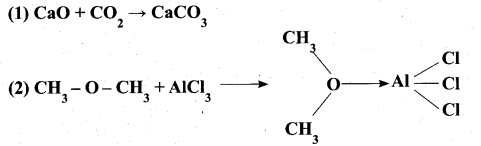(a) (i) 1. Let us consider the reaction between sodium hydroxide and acetic acid to give sodium acetate and water

NaOH(aq) + CH3COOH(aq) ⇌ CH3COONa(aq) + H2O

2. Coordination number of atoms in a bcc structure is 8

(ii) logk = logA – $$\frac{\mathrm{E}_{\mathrm{a}}}{2.303 \mathrm{R}}\left(\frac{1}{\mathrm{T}}\right)$$
y = c + mx
m = $$-\frac{E_{a}}{2.303 R}$$
Ea =—2.303Rm
Ea = – 2.303 x 8.314 J K-1 mol-1 x (— 4000 K)
Ea = 76,589 J mol-1
Ea =76589kJmol-1

[OR]

(b) (i) 1. Coordination number: The number of nearest neighbours that surrounding a particle in a crystal is called the coordination number of that particle.
2. In aqueous solution, CH3COONa is completely dissociated as follows.
CH3COONa(aq), →  CH3COO(aq) + Na+(aq)

3. CH3COO is a conjugate base of the weak acid CHCOOH and it has a tendency to react with H+ from water to produce unionised acid. But there is no such tendency
for Na+ to react with OH

4.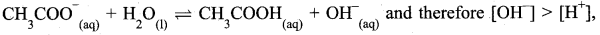such cases, the solution is basic due to the hydrolysis and pH is greater than 7.

5. Relationship between equilibrium constant, hydrolysis constant and the dissociation constant of acid is derived as follows: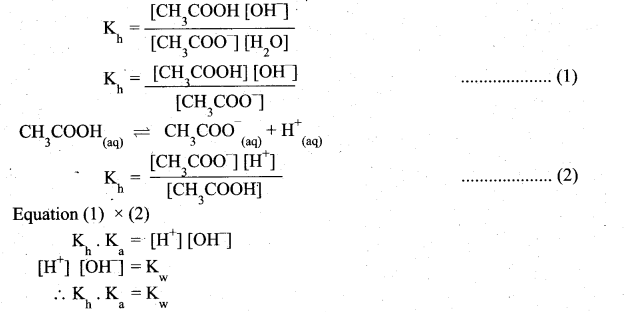Kh value in terms of degree of hydrolysis (h) and the concentration of salt (c) for the equilibrium can be obtained as in the case of Ostwald’s dilution law Kh = h2C and $$\left[\mathrm{OH}^{-}\right]=\sqrt{\mathrm{K}_{\mathrm{h}} \cdot \mathrm{c}}$$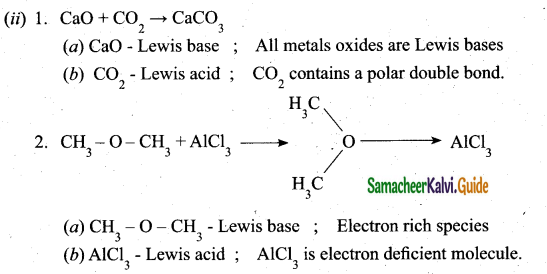Question 37.
(a) (i) What are lyophilic and lyophobic sols? Give one example of each type. Why are
(a) (i) Lyophilic Sols: Colloidal sols directly formed by mixing substances like gums, gelatin, starch, rubber, etc. with a suitable liquid (The dispersion medium) are lyophilic sols.
An important characteristic of these sols is that if the dispersion medium is separated from the dispersed phase (say by evaporation) the sol can be reconstituted by simply remixing with the dispersion medium. That is why these sols are also called reversible sols. These sols are quite stable and cannot be easily coagulated.

Lyophobic sols: These colloidal sols can only be prepared by some special methods. These sols are readily precipitated on the addition of small amount of electrolytes, by heating or by shaking and hence are not stable.
Hydrophobic sols are water hating. They are formed by indirect method. These sols are irreversible sols. These sols are readily precipitated by the addition of small amount of electrolytes, by heating or by shaking and hence are not stable.

(ii) 1. The substances when added to a catalysed reaction decreases or completely destroys the activity of a catalyst are often known as catalytic poisons.
2. In the reaction 2SO2 + O2 → 2SO3 with Pt catalyst, the catalyst poison is AS2O3

[OR]

(b) (i) 1. Substances like AgCl, PbS04 are sparingly soluble in water. The solubility product can be determined using conductivity experiments.
2. Let us consider AgCl as an example
Agci(s)) ⇌ Ag+ + Cl
KSp = [Ag +] [Cl]

3. Let the concentration of [Ag+] be ‘C’ mol L-1
If [Ag+] = C, then [Cl] is also equal to C mol L-1.
.’. Ksp = C.C
Ksp = C2

4. The relationship between molar conductance and equivalent conductance is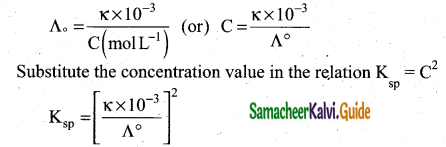(ii) 1. Formic acid contains both an aldehyde as well as an acid group. Hence, like other aldehydes, formic acid can easily be oxidised and therefore acts as a strong reducing agent.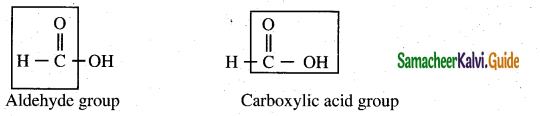2. Formic acid reduces Tollen’s reagent (ammonical silver nitrate solution) to metallic silver.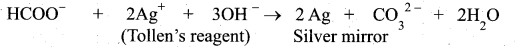3. Formic acid reduces Fehling’s solution. It reduces blue coloured cupric ions to red coloured cuprous ions.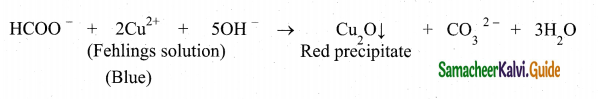Question 38.
(a) (i) What are the uses of nitrobenzene? (2)
(ii) Write a note on formation of -helix. (3)
[OR]
(b) (i) Define TFM value. (2)
(ii) Differentiate thermoplastic and thermosetting. (3)
(a) (i) 1. Nitro benzene is used to produce lubricating oils in motors and machinery.
2. It is used in the manufacture of dyes, drugs, pesticides, synthelic rubber, aniline and explosives like TNT, TNB.
(ii) 1. In the a-helix sub-structure, the aminoacids are arranged in a right handed helical (spiral) structure and are stabilised by the hydrogen bond between the carbonyl oxygen one aminoacid (nth residue) with amino hydrogen of the fifth residue (n+4th residue).

2. The side chains of the residues protrude outside of the helix. Each turn of an a-helix contains about 3.6 residues and is about 5.4 A long.

3. The amino acid pro line produces a line in the helical structure and often called as a helical breaker due to its rigid cyclic structure.

4. Many fibrous proteins such as a-Keratin in hair, nails, wool, skin and myosin in muscles have a-helix structure. Stretching property of human hair is due to the helical structure of a-keratin in hair.

5. Structure of α – helix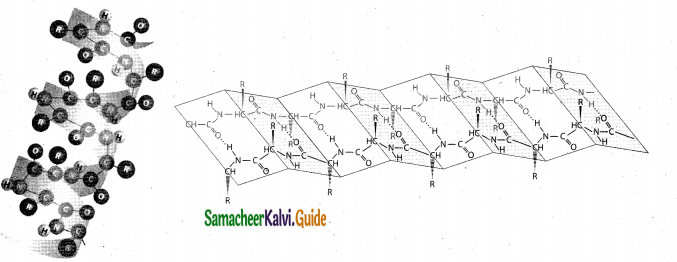[OR]

(b) (i) 1. The quality of a soap is described in terms of total fatty matter (TFM value). It is defined as the total amount of fatty matter that can be separated from a sample after spliting with mineral acids. Higher the TFM value in the soap, better is its quality.
2. As per BIS standards, Grade I soaps should have 76% minimum TFM value (ii) Difference between thermoplastic and thermosetting:

Thermoplastic :

1. They soften on heating and harden on cooling, and they can be remoulded.
2. They consists of linear long chain polymers and low molecular weights polymers.
3. All the polymer chains are held together by weak Vanderwaals forces.
4. They are weak, soft and less brittle.
5. They are formed by addition polymerisation.
6. They are soluble in organic solvents.
7.  Example: PVC, polythene, polystrene etc.

Thermosetting

1. They do not soften on heating and they cannot be remoulded.
2. The consist of three dimensional network structure and high molecular weight polymers.
3. All the polymer chains are linked by strong covalent.
4. They are strong, hard and more brittle.
5. They are formed by condensation polymerisation.
6. They are insoluble in organic solvents.
7. Example: Bakelite, melamine etc.